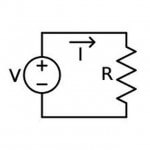Geek Fact for March 16th

In 1787, physicist Georg Simon Ohm was born.Georg Ohm was able to define the fundamental relationship between voltage, current, and resistance. Ohm’s Law states that the amount of steady current (I) through a material is directly proportional to the voltage (V) across the material divided by the electrical resistance (R) of the material, or I = V / R.

Posted in: